# Tamil Nadu 11th Chemistry Model Question Paper 2 English Medium

Students can Download Tamil Nadu 11th Chemistry Model Question Paper 2 English Medium Pdf, Tamil Nadu 11th Chemistry Model Question Papers helps you to revise the complete Tamilnadu State Board New Syllabus and score more marks in your examinations.

## TN State Board 11th Chemistry Model Question Paper 2 English MediumTime: 2½ Hours
Total Score: 70 Marks

General Instructions:

1. The question paper comprises of four parts.
2. You are to attempt all the parts. An internal choice of questions is provided wherever applicable.
3. All questions of Part I. II, III and IV are to be attempted separately.
4. Question numbers 1 to 15 in Part I are Multiple Choice Questions of one mark each. These are to be answered by choosing the most suitable answer from the given four alternatives and writing the option code and the corresponding answer.
5. Question numbers 16 to 24 in Part II are two-mark questions. These are to be answered in about one or two sentences.
6. Question numbers 25 to 33 in Part III are three-mark questions. These are to be answered in about three to five short sentences.
7. Question numbers 34 to 38 in Part IV are five-mark questions. These are to be answered in detail. Draw diagrams wherever necessary.PART-I

Answer all the Questions. [15 x 1 = 1]
Choose the most suitable answer from the given four alternatives.

Question 1.
Match the List-I with List-Il using the correct code given below the list.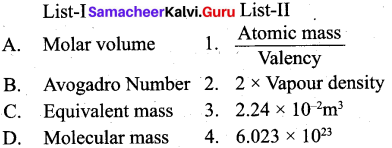Code :
A B C D
(a) 4 3 1 2
(b) 3 4 1 2
(c) 2 1 4 3
(d) 3 1 2 4
(b) 3 4 1 2Question 2.
The energies E1 and E2 of two radiations are 25 eV and 50 eV respectively. The relation between their wavelengths i.e. Xl and X2 will be ……………… .
(a) $$\frac{\lambda_{1}}{\lambda_{2}}=1$$
(b) λ1 = 2λ2
(c) $$\lambda_{1}=\sqrt{25 \times 50} \lambda_{2}$$
(d) 2λ1 = λ2
(b) λ1 = 2λ2

Solution: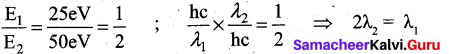Question 3.
In which of the following options the order of arrangement does not agree with the variation of property indicated against it? (NEET 2016 Phase-l)
(a) 1 < Br < Cl < F (increasing electron gain enthalpy)
(b) Li < Na < K < Rb (increasing metallic radius)
(c) AI3+ < Mg2+ < Na+ < F- (increasing ionic size)
(d) B < C < O < N (increasing first ionization enthalpy)
(a) 1 < Br < Cl < F (increasing electron gain enthalpy)Question 4.
For decolourisation of I mole of acidified KMnO4, the moles of H202 required is
(a) 1/2
(b) 3/2
(c) 5/2
(d) 7/2
(c) 5/2

Question 5.
The compound (X) on heating gives a colourless gas and a residue that is dissolved in water to obtain (B). Excess of CO2 is bubbled through aqueous solution of B, C is formed. Solid (C) on heating gives back X. (B) is ………………………. .
(a) CaCO3
(b) Ca(OH)2
(c) Na2CO2
(d) NaHCO3
(b) Ca(OH)2

Solution: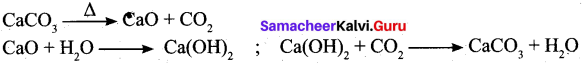Question 6.
Which law is used in the process of enriching the isotope of U235 from other isotopes?
(a) Boyle’s law
(b) Dalton’s law of partial pressure
(c) Graham’s law of diffusion
(d) Charles’ law
(c) Graham’s law of diffusionQuestion 7.
The enthalpies of formation of Al2O3 and Cr2O3 are -1596 kJ and -1134 kJ, respectively. ΔH for reaction 2A1 + Cr2O3 → 2Cr + Al2O3 is …………………… .
(a) -1365 kJ
(b) 2730 kJ
(c) -2730 kJ
(d) -462 kJ
(d) -462 kJ

Solution: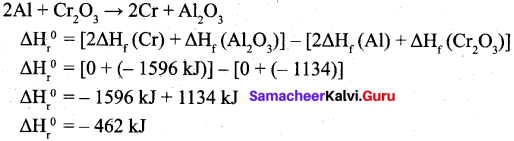Question 8.
Solubility of carbon dioxide gas in cold water can be increased by
(a) increase in pressure
(b) decrease in pressure
(c) increase in volume
(d) none of these
(a) increase in pressureSolution: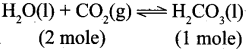increase in pressure, favours the forward reaction.

Question 9.
Two liquids X and Y on mixing gives a warm solution. The solution is
(a) ideal
(b) non-ideal and shows positive deviation from Raoults law
(c) ideal and shows negative deviation from Raoults Law
(d) non-ideal and shows negative deviation from Raoults Law
(d) non-ideal and shows negative deviation from Raoults Law

Solution: ΔHmix is negative and show negative deviation from Raoults law.

Question 10.
Which of the following molecules have bond order equal to 1?
(a) NO, HF, HC1, Li2, CO
(b) H2, Li2, HF, Br2, HC1
(c) Li2, B2, CO, NO, He2+
(d) B2, CO, He2+, NO, HF
(b) H2, Li2, HF, Br2, HC1Question 11.
How many cyclic and acyclic isomers are possible for the molecular formula C3H60?
(a) 4
(b) 5
(c) 9
(d) 10
(c) 9

Question 12.
Statement-I: Fluoro acetic acid is stronger acid than acetic acid
Statement-II: Fluorine has high electronegativity and it is facilitate to dissociate the O-H bond easily.
(а) Statement-I and II are correct and statement-II is correct explanation of statement-I.
(b) Statement-I and II are correct but statement-II is not correct explanation of statement-I.
(c) Statement-I is correct but statement-II is wrong.
(d) Statement-I is wrong but statement-II is correct
(а) Statement-I and II are correct and statement-II is correct explanation of statement-I.

Question 13.
Consider the following statements.
(i) Alkanes are saturated hydrocarbons in which all the bonds between the carbon atoms are single bond.
(ii) Alkenes are saturated hydrocarbons in which atleast one carbon – carbon double bond is present.
(iii) Alkynes are unsaturated hydrocarbons in which atleast one carbon – carbon triple bond is present.
Which of the above statement is/are correct?
(a) (i) and (ii)
(b) (i) and (iii)
(c) (ii) and (iii)
(d) only (ii)
(b) (i) and (iii)Question 14.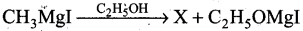Product X is ……………….. .
(a) CH4
(b) C2H6
(c) HCHO
(d) CH3OH
(a) CH4

Question 15.
Eutrophication causes reduction in
(a) dissolved oxygen
(b) dissolved nitrogen
(c) dissolved salts
(d) all of the above
(a) dissolved oxygen

Part-II

Answer any six questions in which question No. 22 is compulsory. [6 x 2 = 12]Question 16.
Explain about the factors that affect electronegativity?

• Effective nuclear charge: As the nuclear charge increases, electronegativity also increases along the periods.
• Atomic radius: The atoms in smaller size will have larger electronegativity.

Question 17.
How do you convert para-hydrogen into ortho-hydrogen?
Para hydrogen can be converted into ortho hydrogen by the following ways:

• By treating with catalysts platinum or iron.
• By passing an electric discharge.
• By heating > 800°C.
• By mixing with paramagnetic molecules such as O2, NO, NO2.
• By treating with nascent/atomic hydrogen.

Question 18.
Gases don’t settle at the bottom of a container?
Gases by definition are the least dense state of matter. They have negligible intermolecular forces of attraction. So they are all free to roam separately. So the least dense gas particles will not sink at the bottom of a container.Question 19.
Define – Normality.
Normality (N): It is defined as the number of gram equivalents of solute in 1 litre of the solution.
$$\mathrm{N}=\frac{\text { Number of gram equivalent of solute }}{ \text { Volume of solution (in }\mathrm{L})}$$

Question 20.
If there is no change in concentration why is the equilibrium state considered dynamic?
At chemical equilibrium the rate of two opposing reactions are equal and the concentration of reactants and products do not change with time. This condition is not static and is dynamic, because both the forward and reverse reactions are still occurring with the same rate and no macroscopic change is observed. So chemical equilibrium is in a state of dynamic equilibrium.

Question 21.
In CH4, NH3 and H20 the central atom undergoes sp3 hybridization – yet their bond angles are different. Why?

1. In CH4, NH2 and H2O the central atom undergoes sp3 hybridisation. But their bond angles are different due to the presence of lone pair of electrons.
2. It can be explained by VSEPR theory. According to this theory, even though the hybridisation . is same, the repulsive force between the bond pairs and lone pairs are not same.
3. Bond pair-Bond pair < Bond pair — Lone pair < Lone pair -Lone pair
So due to the varying repulsive force the. bond pairs and lone pairs are distorted from regular geometry and organise themselves in such a way that repulsion will be minimum and stability will be maximum.
4. In case of CH4, there are 4 bond pairs and no lone pair of electrons. So it remains in its regular geometry, i.e., tetrahedral with bond angle = 109° 28’.
5. H2O has 2 bond pairs and 2 lone pairs. There is large repulsion between Ip-lp. Again repulsion between Ip-bp is more than that of 2 bond pairs. So 2 bonds are more restricted to form inverted V shape (or) bent shape molecule with a bond angle of 104° 35’.
6. NH3 has 3 bond pairs and 1 lone pair. There is repulsion between Ip-bp. So 3 bonds are more restricted to form pyramidal shape with bond angle equal to 107° 18’.Question 22.
Identify the compound in the following reaction,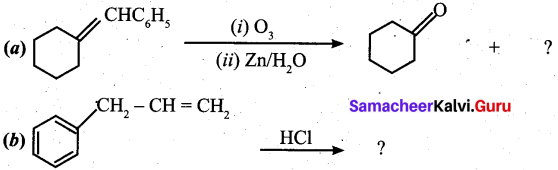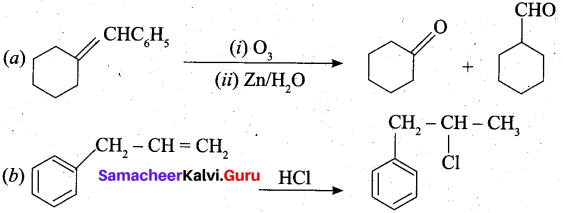Question 23.
ClassIfy the following compounds in the form of alkyl, allylic, vinyl, benzyllc halides,
(a) CH2 = CH – CH2 CI
(b) C6H5CH2I
(c) CH3 – CH – CH3
(d) CH2 = CH – Cl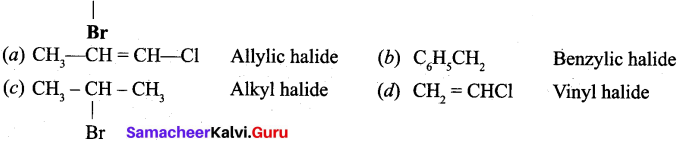Question 24.
Define — Smog.

1. Smog is a combination of’ smoke and fog which form droplets that remains suspended in the air.
2. Smog is a chemical misture of gases that forms a brownish yellow haze. It mainly consists of ground level ozone, oxideš of nitrogen, volatile organic compounds, SO2, acidic aerosols and some other gases.Part – III

Answer any six questions in which question No. 30 is compulsory. [6 x 3 = 18]

Question 25.
Explain how effective nuclear charge is related with stability of the orbital?

1. In a multi-electron atom, in addition to the electrostatic attractive force between the electron and nucleus, there exists a repulsive force among the electrons.
2. These two forces are operating in the opposite direction. This results in the decrease in the nuclear force of attraction on electron.
3. The net charge experienced by the electron is called effective nuclear charge.
4. The effective nuclear charge depends on the shape of the orbitals and it decreases with increase in azimuthal quantum number.
5. The order of the effective nuclear charge felt by a electron in an orbital within the given shell is s > p > d > f.
6. Greater the effective nuclear charge, greater is the stability of the orbital. Hence, within a given energy level, the energy of the orbitals are in the following order s < p < d < f.Question 26.
Write a notes on hydrogen sponge.
(i) Hydrogen sponge (or) Metal hydride e.g., palladium-hydrogen system is a binary hydride (Pdll).
(ii) Upon heating, H atoms diffuse through the metal to the surface and recombine to form molecular hydrogen. Since no other gas behaves this way with palladium, this process has been used to separate hydrogen gas from other gases:
$$2 \mathrm{Pd}_{(\mathrm{s})}+\mathrm{H}_{2(\mathrm{g})} \rightleftharpoons 2 \mathrm{PdH}_{(\mathrm{s})}$$
(iii) The hydrogen molecule readily adsorb on the palladium surface, where it dissociates into atomic hydrogen. The dissociated atoms, dissolve into the interstices or voids (octahedral or tetrahedral) of the crystal lattice.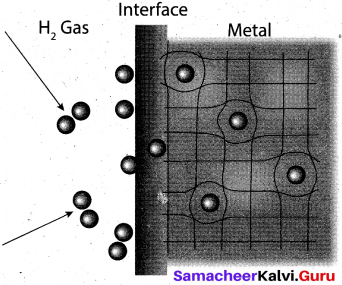(iv) Technically, the formation of metal hydride is by chemical reaction but it behaves like a physical storage method, i. e., it is absorbed and released like a water sponge. Such a reversible uptake of hydrogen in metals and alloys is also attractive for hydrogen storage and for rechargeable metal hydride battery applications.Question 27.
In what way lithium differs from other metals of the same group?

 Lithium Other elements of the family Very hard. Very Soft. High melting and boiling point. Low melting and boiling point. Least reactive. More reactive. Reacts with nitrogen to get Li3N. No reaction. Reacts with bromine slowly. Reacts violently. Burnt in air gives monoxide only.Burnt in air gives peroxides also, apart from monoxides. K, Rb and Cs gave superoxides. Compounds are partially soluble in water. Highly soluble in water. Lithium nitrate decomposes to form an oxide. Other metals on heating gives nitrite. Extremely small in size. Comparatively large in size. Li+ has greater polarizing power. Other M+ ions have comparatively larger polarizing power.

Question 28.
A 0.25 M glucose solution at 370.28 K has approximately the pressure as blood does what is the osmotic pressure of blood?
Sol.
C = 0.25 M
T = 370.28 K
(π)glucose = CRT
(π) = 0.25 mol L-1 x 0.082 L atm K-1 mol-1 x 370.28K
= 7.59 atm

Question 29.
Write the important principles of VSEPR theory to predict the shape of molecules.

1. The shape of a molecule depends upon the no. of electron pairs around the central atom.
2. There is a repulsive force between the electron pairs, which tend to repel one another.
3. The electron pairs in space tend to occupy such positions that they are at maximum distance, so that the repulsive force will be minimum.
4. A multiple bond is treated as if it is a single bond and the remaining electron pairs which constitute the bond may be regarded as single super pair.Question 30.
0.26 g of an organic compound gave 0.039 g of water and 0.245 g of CO2 on combustion. Calculate the percentage of carbon and hydrogen.
Weight of organic compound = 0.26 g
Weight of water = 0.039 g
Weight of CO2 = 0.245 g

Percentage of hydrogen
18 g of water contains 2 g of hydrogen
0.039 g of water contains = $$\frac{2}{18} \times \frac{0.039}{0.26} \text { of } \mathrm{H}$$
∴ % of hydrogen = $$\frac{0.039}{0.26} \times \frac{2}{18}$$ x 100 = 1.66%

Percentage of carbon
44 g of CO2 contains 12 g of C
0. 245 g of CO2 contains = $$\frac{12}{44} \times \frac{0.245}{0.26}$$ g of C
% of Carbon = $$\frac{12}{44} \times \frac{0.245}{0.26}$$ x 100 = 25.69%

Question 31.
How will you distinguish l-butyne and 2-butyne?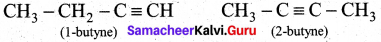In l-butyne, terminal carbon atom contains atom one acidic hydrogen, therefore it will react with silver nitrate in the presence of ammonium hydroxide to give silver butynide. Whereas 2-butyne does not undergo such type of the reaction, because of the absence of acidic hydrogen.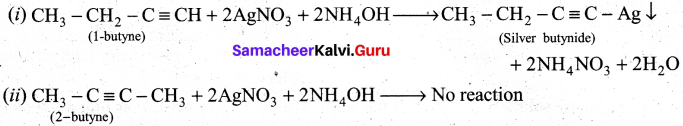Question 32.
Write the structure of the following compounds,
(a) l-Bromo-4 -ethyl cyclohexane
(b) 1, 4-Dichlorobut-2-ene
(c) 2-Chloro-3-methyl pentane
(a) l-bromo-4 ethylcyclo hexane
(b) 1,4-dichloro but-2-ene
(c) 2-chloro-3-methyl pentane Br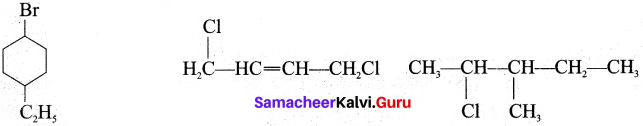Question 33.
Explain how does green house effect cause global warming.

1. The earth’s atmosphere allows most of the visible light from the sun to pass through and reach the earth’s surface. As earth’s surface is heated by sunlight, it radiates a part of this energy back towards the space as longer IR wavelengths.
2. Some of the heat is trapped by CH4, CO2, CFCs and water vapour present in the atmosphere. They absorb IR radiations and block a large portion of earth’s emitted radiations.
3. The radiations thus absorbed is partly remitted to the earth’s surface. Therefore the earth’s surface gets heated up by a phenomenon called greenhouse effect.
4. Thus greenhouse effect is defined as the heating up of the earth surface due to trapping of infrared radiations reflected by earth’s surface by CO2 layer in the atmosphere. The heating up of the earth through the greenhouse effect is called global warming.

Part-IV

Answer all the questions. [5 x 5 = 25]

Question 34.
(a) (i) Write the steps to be followed for writing empirical formula. (3)
(ii) What do you understand by the terms empirical formula and molecular formula? (2)
[OR]
(b) (i) Explain the shape of s and p-orbital. (2)
(ii) The mass of an electron is 9.1 x 10-31 kg. If its kinetic energy is 3.0 x 10-25 J. Calculate its wavelength. (3)
(a) (i) Empirical formula shows the ratio of numher of atoms of different elements in one molecule of the compound.Steps for finding the Empirical formula:
The percentage of the elements in the compound is determined by suitable methods and from the data collected; the empirical formula is determined by the following steps.

1. Divide the percentage of each element by its atomic mass. This will give the relative number of atoms of various elements present in the compound.
2. Divide the atom value obtained in the above step by the smallest of them so as to get a simple ratio of atoms of various elements.
3. Multiply the figures so obtained, by a suitable integer if necessary in order to obtain whole number ratio.
4. Finally write down the symbols of the various elements side by side and put the above numbers as the subscripts to the lower right hand of each symbol. This will represent the empirical formula of the compound.
5. Percentage of Oxygen = 100 – Sum of the percentage masses of all the given elements.

(ii)

 Empirical Formula Molecular Formula It is the simplest formula. It is the actual formula. It shows the ratio of number of atoms of different elements in one molecule of the compound. It shows the actual number of different types of atoms present in one molecule of the compound.

(b) (i) s-orbital: For is orbital, l = 0, m = 0, f(θ) = 1/√2 and g((φ) = 1√2π. Therefore, the angular distribution function is equal to 1/2√π. i.e. it is independent of the angle θ and φ. Hence, the probability of finding the electron is independent of the direction from the nucleus. So, the shape of the s orbital is spherical.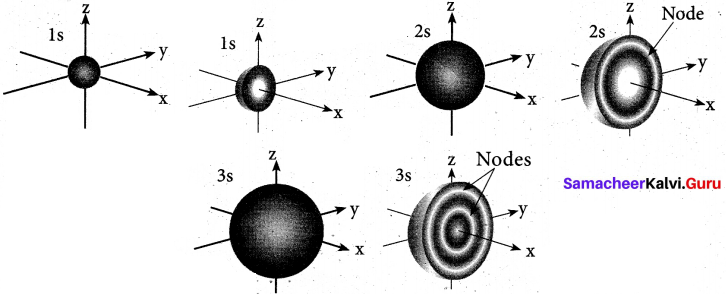p-orbital: For p orbitals l = 1 and the corresponding m values are -1, 0 and +1. The three different m values indicates that there are three different orientations possible for p orbitals. These orbitals are designated as px, py and pz. The shape of p orbitals are dumb bell shape.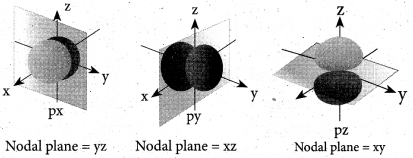(ii) Step I. Calculation of the velocity of electron
Kinetic energy =1/2 mu2 = 3.0 x 10-25 J = 3.0 x 10-25 kg m2 s-2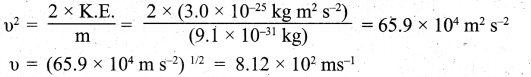Step II. Calculation of wavelength of the electron According to de Broglie’s equation,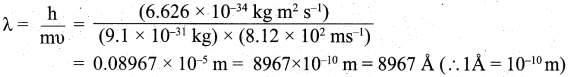Question 35.
(a) (i) Explain the exchange reactions of deuterium, (2)
(ii) Explain the action of soap with hard water. (3)
[OR]
(b) (i) Derive ideal gas equation. (3)
(ii) CO2 gas cannot be liquified at room temperature. Give the reason. (2)
(a) (i) Deuterium can replace reversibly hydrogen in compounds either partially or completely depending upon the reaction conditions. These reactions occur in the presence of deuterium.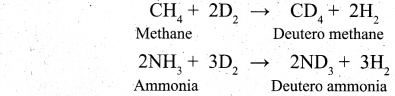(ii) Action of soap with hard water:

• The cleaning capacity of soap is reduced when used in hard water.
• Soaps are sodium or potassium salts of long chain fatty acids.
• When soap is added to hard water, the divalent magnesium and calcium cations present in hard water relict with soap.
• The sodium salts present in soaps are converted to their corresponding magnesium and calcium.salts which are precipitated as scum or precipitate.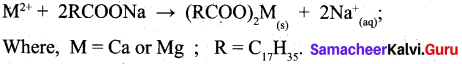(b) (i) Ideal gas equation:
The gaseous state is described completely using the following four variables T, P, V and n. Each gas law relates one variable of a gaseous sample to another while the other two variables are held constant.

Therefore, combining all equations into a single equation will enable to account for the change in any or all of the variables.

Boyle’s law: V α $$\frac{1}{P}$$
Charles’ law: V α T

We can combine these equations into the following general equation that describes the physical behaviour of all gases.
$$\mathrm{V} \propto \frac{\mathrm{nT}}{\mathrm{P}}$$

The above equation can be rearranged to give PV = nRT – Ideal gas equation.

Where, R is also known as Universal gas constant.
(ii) Only below the critical temperature, by the application of pressure, a gas can be liquefied. CO2 has critical temperature as 303.98 K. Room temperature means (30 + 273 K) 303 K. At room temperature, (critical temperature) even by applying large amount of pressure CO2 cannot be liquefied. Only below the critical temperature, it can be liquefied. At room temperature, CO2 remains as gas.Question 36.
(a) (i) Why deep-sea divers use air diluted with helium gas in their air tanks? (3)
(ii) What is molal depression constant? Does it depend on nature of the solute. (2)

[OR]

(b) (i) Write the resonance structures for ozone molecule and N20? (2)
(ii) Draw MO diagram of CO and calculate its bond order. (3)
(a) (i) 1. Deep-sea divers carry a compressed air tank for breathing at high pressure under water. This air tank contains nitrogen and oxygen which are not very soluble in blood and other body fluids at normal pressure.
2. As the pressure at the depth is far greater than the surface atmospheric pressure, more nitrogen dissolves in the blood when the diver breathes from tank.
3. When the divers ascends to the surface, the pressure decreases, the dissolved nitrogen comes out of the blood quickly forming bubbles in the blood stream. These bubbles restrict blood flow, affect the transmission of nerve impulses and can even burst the capillaries or block them. This condition is called “the bends” which are painful and dangerous to life.
4. To avoid such dangerous condition they use air diluted with helium gas (11.7 % helium, 56.2% nitrogen and 32.1% oxygen) of lower solubility of helium in the blood than nitrogen.

(ii) Kf = molar freezing point depression constant (or) cryoscdpic constant. ΔTf = Kf. m, where ΔTf = depression in freezing point, m = molality of the solution Kf= cryoscopic constant If m = 1, ΔTf = kfi. e., cryoscopic constant is equal to the depression in freezing point for 1 molal solution cryoscopic constant depends on the molar concentration of the solute particles. Kf is directly proportional to the molal concentration of the solute particles.
$$\Delta \mathrm{T}_{f}=\mathrm{K}_{f} \times \frac{\mathrm{W}_{\mathrm{B}} \times 1000}{\mathrm{M}_{\mathrm{B}} \times \mathrm{W}_{\mathrm{A}}}$$

WB = mass of the solute, WA = mass of solvent, MB = molecular mass of the solute.

[OR]

(b)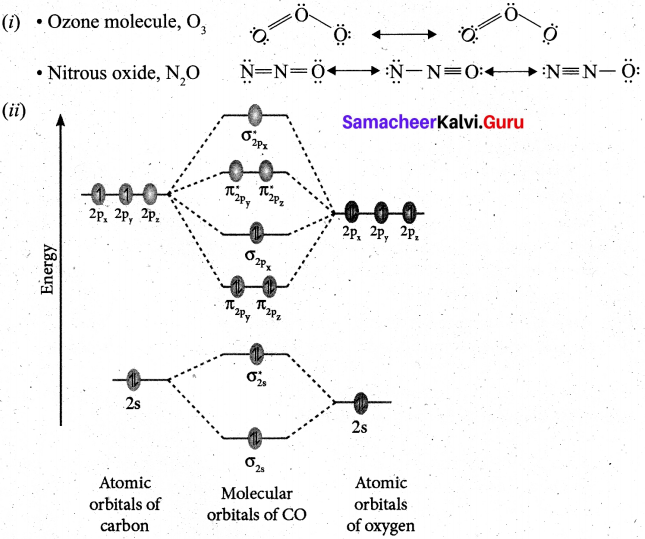• Electronic configuration of C atom: Is2, 2s2 2p2
Electronic configuration of O atom: ls2 2s2 2p4
• Electronic configuration of CO molecule is: $$\sigma 1 s^{2} \sigma^{*} 1 s^{2} \sigma 2 s^{2} \sigma^{*} 2 s^{2} \pi 2 p_{y}^{2} \pi 2 p_{z}^{2} \sigma 2 p_{x}^{2}$$
• Bond order = $$\frac{N_{b}-N_{a}}{2}=\frac{10-4}{2}=3$$
• Molecule has no unpaired electron, hence it is diamagnetic.

Question 37.
(a) (i) What are cis-trans isomerism? Explain with example.
(ii) Give the IUPAC names of the following compounds,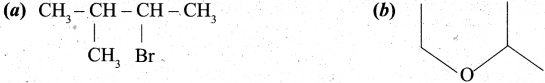[OR]

(b) (i) What is enzymatic browning?
(ii) How will you convert nitrile into primary amine?
(a) (i)

• Geometrical isomers are the stereoisomers which have different arrangement of groups or atoms around a rigid framework of double bonds. This type of isomerism occurs due to restricted rotation of double bonds or about single bonds in cyclic compounds.
• In 2-butene, the carbon-carbon double bond is sp2 hybridised. The carbon-carbon double bond consists of a a bond and a n bond. The presence of π bond lock the molecule in one position. Hence, rotation around C = C bond is not possible.
•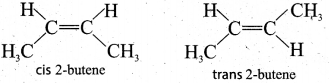• These two compounds are termed as geometrical isomers and are termed as cis and trans form.
• The cis isomer is the one in which two similar groups are on the same side of the double bond. Thetrans isomer is that in which two similar groups are on the opposite side of the double bond. Hence, this type of isomerism is called cis-trans isomerism.(ii)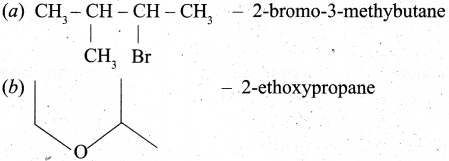[OR]

(b) (i) 1. Apples contains an enzyme called polyphenol oxidase (PPO) also known as tyrosinase.
2. Cutting an apple exposes its cells to the atmospheric oxygen and oxidizes the phenolic compounds present in apples. This is called the “enzymatic browning” that turns a cut apple brown.
3. In addition to apples, enzymatic browning is also evident in bananas, pears, avocados and even potatoes.

(ii) Converting nitrile into primary amine: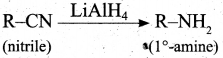Question 38.
(a) An organic compound (A) of a molecular formula C2H4, decolourises bromine water. (A) on reacts with Cl2 gives (B). (A) reacts with HBr to give (C). (A) reacts with hydrogen in the presence of Ni to give D. Identify A, B, C and D. Explain the reactions. (5)[OR]

(b) (i) Explain the harmful effects of acid rain. (3)
(ii) What is Eutrophication? (2)
(a) 1. C2H4 (A), decolorises the bromine water. Therefore it contains double bond. Hence (A) is ethylene.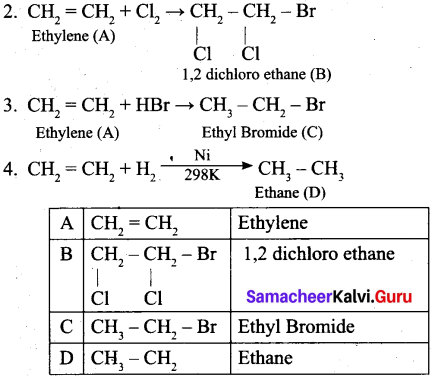[OR]

(b) (i)

1. Acid rain causes damage to buildings made us of marbles. This attack on marble is termed as stone leprosy.
CaCO3 + H2SO4 → CaSO4 + H2O + CO2
2. Acid rain affects plant and animal life in aquatic ecosystem.
3. It is harmful for agriculture, as it dissolves in the earth and removes the nutrients needed for the growth of plants.
4. It corrodes water pipes resulting in the leaching of heavy metals such as iron, lead and copper into drinking water which have toxic effects.
5. It causes respiratory ailment in humans and animals.(ii) Eutrophication: When the growth of algae increases in the surface of water, dissolved oxygen in water is greatly reduced. This phenomenon is known as eutrophication. Due to this growth of fishes gets inhibited.